# 1.- Introduction

In this essay I'm going to propose two possible three-dimensional structures of the Fruit of Life. Each one is supported on a particular 3D inner grid that is contained into the inner grid of Metatron's Cube. This was a necessary condition for me, as the Fruit of Life is contained into the Flower of Life and the latter defines the Cube.

Remember that in 2D the Fruit of Life is constructed after completing two outer layers of circles in the Flower of Life until obtaining three lines of five nonintersecting circles as shown in the following figure:Figure 1: The accepted two dimensional form of the Fruit of Life

# 3.- First 3D structure: a cube of 3x3x3 spheres

The two 3D structures that I propose require the completion of the remaining six circles in the 2D representation of the Fruit of Life (Figure 2):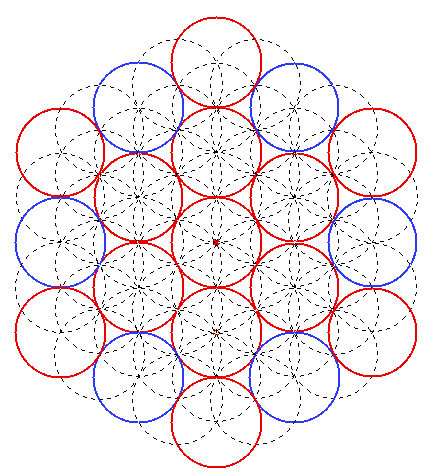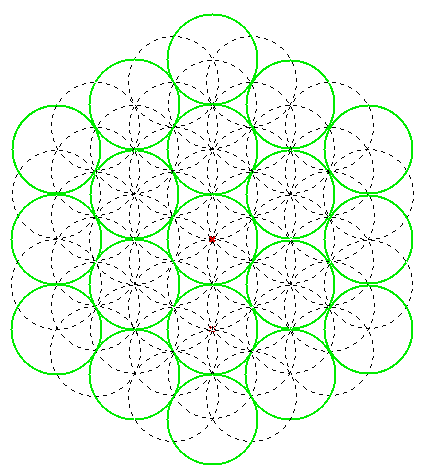Figure 2: Completion of the remaining non-overlapping circles of the 2D Fruit of Life. The final figure has 19 circles.

At first sight the projection in Figure 2 can be extended to three dimensions as a cube of 3x3 spheres per side, totaling 27 spheres (Figure 3). The final result would be similar to Metatron's cube, but with a sphere less per edge:Figure 3: First three dimensional structure proposed for the Fruit of Life. You can click on the image to see a true 3D model that you can zoom, rotate and pan.

This structure of spheres is clearly contained in Metatron's Cube. Coming from the 3D structure of the Egg of Life as a cube made of 2x2x2 spheres, the next step in a fractal growth would be the Fruit of Life as a cube made of 3x3x3 spheres, and the final step a cube made of 4x4x4 spheres that I claim to be Metatron's Cube. This process can be illustrated using the same XY projection of the grid coordinates from Metatron's Cube:Figure 4:  A natural growth process starting with the Egg of Life (a cube made of 2x2x2=8 spheres), going through the Fruit of Life as a cube made of 3x3x3=27 spheres, and ending up in Metatron's Cube containing 4x4x4=64 spheres.

The inner structue that supports the cube made of 3x3x3 spheres is not evident. You can obtain it by retaining any corner of size 3x3 from the inner grid of Metatron's Cube. The final result is the grid shown in Figure 5. You can see in that this grid has a big tetrahedron intersecting a set of four cuboctahedrons (actually vector equilibrium structures). These are distributed as one line made of two cuboctahedrons side by side, on top of another identical line rotated 90 degrees.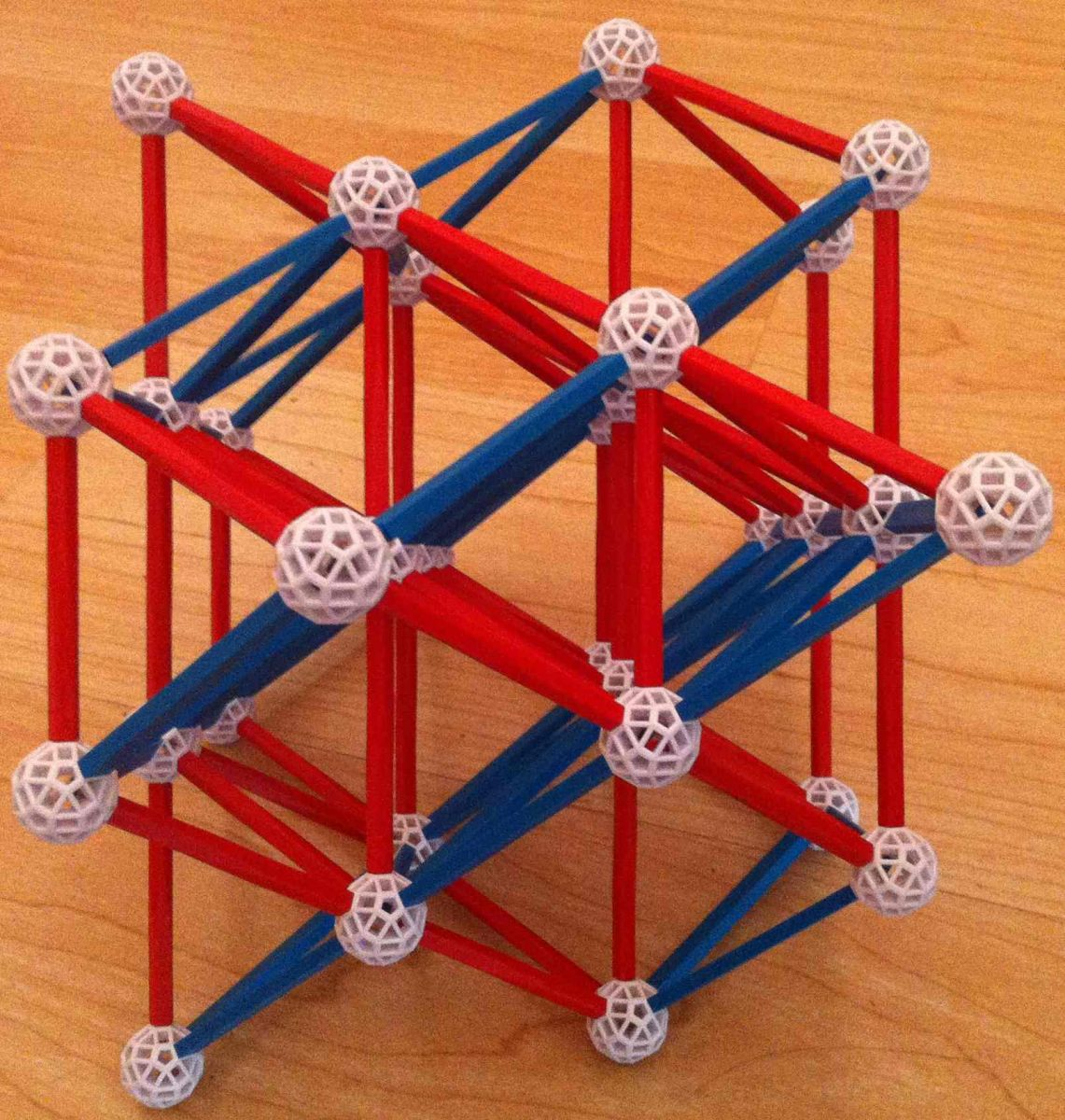Figure 5: The inner grid that supports the first three dimensional structure proposed for the Fruit of Life. The grid contains a big tetrehedron and four vector equilibrium structures.

The 27 spheres that form the 3D structure of the Fruit of Life can be tought of the 26 facets of a cube, plus a central sphere: the 6 faces are represented by the 6 central spheres in each side; the 8 vertices would be the 8 corner spheres; and the 12 edges are the remaining 12 outer spheres joining each couple of vertices.

This cube made of 3x3x3=27 spheres admits an interpretation in terms of the trigrams. The trigrams are groups of three elements taken from three possible base essences: the Tao (a dot), the Yin (a line) and the Yang (a dotted line). This makes a total of 27 possible combinations. The trigrams can be assigned to these 26+1 facets of the cube in the form proposed by James Barton (Figure 6). The central sphere would correspond to the Tao; the spheres in the faces, to the 6 trigrams with two Tao elements; the spheres in the edges to the 12 trigrams with one Tao element; and the corner spheres to the 8 trigrams with only Yin and Yang. It is worth noting at this point that the set of 27 trigrams can be linked to the set of 64 hexagrams, which in turn coincide with the number of spheres in Metatron's Cube.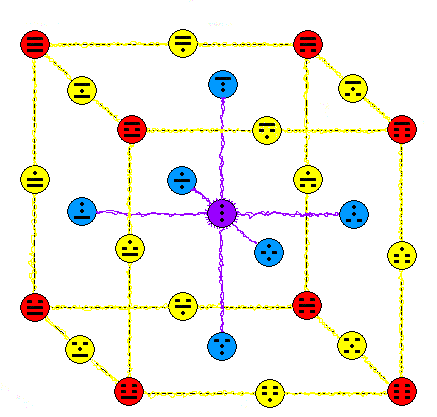Figure 6:  The distribution of the 27 trigrams in the 26+1 facets of a cube proposed by James Barton.

# 3.- Second 3D structure: a cuboctahedron of 55 spheres

The augmented 2D Fruit of Life shown in Figure 2 can also be thought as the projection of a three dimensional set of spheres distributed as a cuboctahedron instead of a cube (Figure 7). It is the big cuboctahedron contained inside the grid of Metatron's Cube. In this case, the spheres would occupy the vertices of the grid, instead of being located at the center of the small tetrahedrons defined by the grid (that's why we draw them in red instead of green). As explained in the article on Metatron's Cube, this grid can be seen as grown out from the central cuboctahedron (actually vector equilibrium) by a process of doubling the edge size. It is a fractal growth also in another sense: the central cuboctahedron contains 13 vertices, whereas the big cuboctahedron contains 13 smaller cuboctahedrons.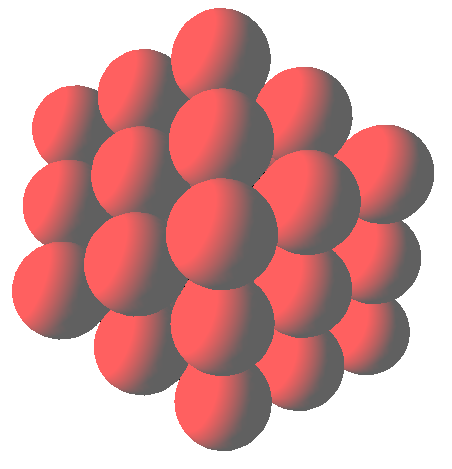Figure 7:  The second 3D possible structure proposed for the Fruit of Life.

I don't know whether any of these two structures is the actual three dimensional one behind the Fruit of Life. Both of them can be found projected in the pattern of the Flower of Life, and also in the 3D pattern of Metatron's Cube. If you have any feedback concerning any of these proposals please let me know!